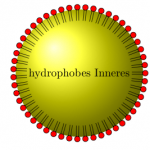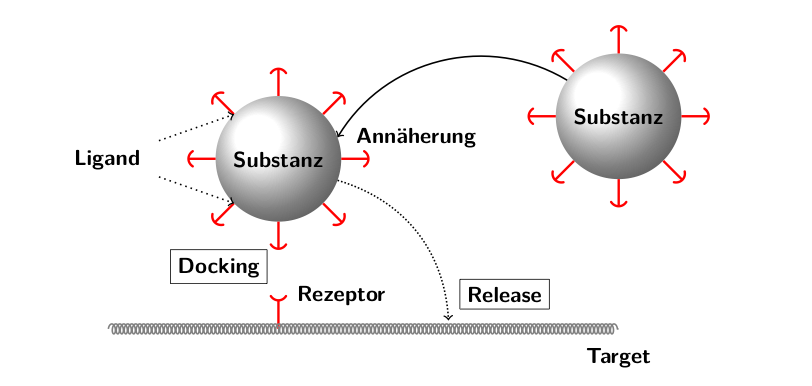# Liposomen und Vesikel

Einige Bilder in TikZ… Ein Solidlipid Nanoparticle…und ein paar Prinzipien:% % path Decoration by Henrik Skov Midtiby, altered, see http://www.texample.net/tikz/examples/lipid-vesicle/ This is needed for the two Vesicles w surfactants \documentclass{report} \usepackage{tikz} \usetikzlibrary{arrows,positioning, trees, decorations,patterns, decorations.pathmorphing, decorations.pathreplacing,shapes.misc} %%%%%% \pgfdeclaredecoration{lipidleaflet}{initial} { % Place as many segments as possible along the path to decorate % the minimum distance between two segments is set to 7 pt. \state{initial}[width=\pgfdecoratedpathlength/floor(\pgfdecoratedpathlength/7pt)] { % Draw the two acyl chains \pgfpathmoveto{\pgfpoint{-1pt}{0pt}} \pgfpathlineto{\pgfpoint{-1pt}{-10pt}} \pgfpathmoveto{\pgfpoint{1pt}{0pt}} \pgfpathlineto{\pgfpoint{1pt}{-10pt}} % Draw the head group \pgfpathmoveto{\pgfpoint{1pt}{0pt}} \pgfpathcircle{\pgfpoint{0pt}{2pt}}{2.5pt} % \pgfpathmoveto{\pgfpoint{0pt}{4.5pt}} \pgfpathlineto{\pgfpoint{0pt}{7pt}} \pgfpathmoveto{\pgfpoint{0pt}{7pt}} \pgfpathlineto{\pgfpoint{1pt}{8pt}} \pgfpathmoveto{\pgfpoint{1pt}{8pt}} \pgfpathlineto{\pgfpoint{-1pt}{9pt}} \pgfpathmoveto{\pgfpoint{-1pt}{9pt}} \pgfpathlineto{\pgfpoint{2pt}{10pt}} % } \state{final} { \pgfpathmoveto{\pgfpointdecoratedpathlast} } }   \pgfdeclaredecoration{lipidleaflet2}{initial} { % Place as many segments as possible along the path to decorate % the minimum distance between two segments is set to 7 pt. \state{initial}[width=\pgfdecoratedpathlength/floor(\pgfdecoratedpathlength/7pt)] { % Draw the two acyl chains \pgfpathmoveto{\pgfpoint{-1pt}{0pt}} \pgfpathlineto{\pgfpoint{-1pt}{-10pt}} \pgfpathmoveto{\pgfpoint{1pt}{0pt}} \pgfpathlineto{\pgfpoint{1pt}{-10pt}} % Draw the head group \pgfpathmoveto{\pgfpoint{1pt}{0pt}} \pgfpathcircle{\pgfpoint{0pt}{2pt}}{2.5pt} } \state{final} { \pgfpathmoveto{\pgfpointdecoratedpathlast} } } \begin{document} % SLN \begin{tikzpicture}[scale=1,every node/.style={font=\small, text centered}] \draw[decorate, decoration={lipidleaflet2}] (0, 0.5) circle (1.2cm); \shade[shade,ball color=yellow] (0, 0.5) circle (2cm); \draw[decorate, decoration={lipidleaflet2, mirror},fill=red] (0, 0.5) circle (2cm); %\draw (5, -3.5) node[text width=4 cm] {Solid Lipid Nanoparticle}; \draw (0,0.5) node {hydrophobes Inneres}; \end{tikzpicture}   % Liposomes % unilammelar (A) and multilamllar Liposome (B) \begin{tikzpicture}[every node/.style={font=\small, text width=2 cm, text centered}]   \shade[shade,ball color=blue!20] (5, 0.5) circle (1.2cm); \draw[decorate, decoration={lipidleaflet2},fill=red] (5, 0.5) circle (1.2cm); \draw[decorate, decoration={lipidleaflet2, mirror},fill=red] (5, 0.5) circle (2cm);   \draw (5,0.5) node {hydrophiles Inneres};   \draw (5,5) node[font=\bfseries] {A}; \draw (11,5) node[font=\bfseries] {B};   \shade[shade,ball color=blue!20] (11, 0.5) circle (1.2cm); \draw[decorate, decoration={lipidleaflet2},fill=red] (11, 0.5) circle (1.2cm); \draw[decorate, decoration={lipidleaflet2, mirror},fill=red] (11, 0.5) circle (2cm); \draw[decorate, decoration={lipidleaflet2},fill=red] (11, 0.5) circle (2.4cm); \draw[decorate, decoration={lipidleaflet2, mirror},fill=red] (11, 0.5) circle (3.2cm);   \draw (11,0.5) node {hydrophiles Inneres}; \end{tikzpicture} % general behaviour and mechanisms of small vesicles   \begin{tikzpicture}[% scale=1.5, pin distance=5mm, every pin edge/.style={very thick,-),red}, initial/.style={circle,inner sep=0.25cm, thick,pin=left: ,pin=right: ,pin=above: , pin=below: ,pin= above right:, pin=above left: , pin=below right: ,pin=below left:, shade,ball color=white,outer sep=0mm }, every node/.style={font=\sffamily\bfseries,black, outer sep=2mm} ] \node at (5,2.5)[initial](come) {Substanz}; \node at (1,2) [initial](par) { Substanz}; \draw[-&gt;,thick] (come.145) to [bend right=45] (par.20) node[ right ]{aproach} ; \draw [densely dotted,thick,-&gt;, ] (par.340) to [bend left=35] (3,0.1) node[above right,draw=black, solid,thin] {Release}; \draw [ very thick,-(,red] (1,0) to (1,0.4) node[above left,draw=black,thin]{Docking} node[right]{Rezeptor} ; \draw[decorate,decoration={coil,segment length=2pt}, thick,color=black!50] (-1,0) -- (5,0) node[below]{Target}; \node (ligand) [left = of par] {Ligand}; \draw[dotted,thick,-&gt;] (ligand) to (par.225); \draw[dotted,thick,-&gt;] (ligand) to (par.135); \end{tikzpicture}   \end{document}
Dieser Beitrag wurde unter (La)TeX abgelegt und mit , verschlagwortet. Setze ein Lesezeichen auf den Permalink.

Die Kommentarfunktion ist geschlossen.